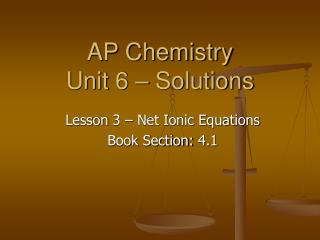# AP Chemistry Unit 6 – Solutions - PowerPoint PPT PresentationDownload PresentationAP Chemistry Unit 6 – Solutions

Presentation Description
Download Presentation## AP Chemistry Unit 6 – Solutions

- - - - - - - - - - - - - - - - - - - - - - - - - - - E N D - - - - - - - - - - - - - - - - - - - - - - - - - - -
##### Presentation Transcript

1. AP ChemistryUnit 6 – Solutions Lesson 3 – Net Ionic Equations Book Section: 4.1

2. Oxidation Number • This is something I should have covered much earlier, but I planned to do it now… so here goes. • The oxidation number of an element in a compound is its degree of oxidation in a compound. The greater the number, the more oxidized it is. • We have used common oxidation numbers for s and p block elements.

3. Oxidation Number • Rules: • Alkali metals are +1 • Alkaline earth metals are +2 • Oxygen is usually -2, unless it is a peroxide (-1) • Hydrogen is +1 • Total oxidation must equal total charge • Example: • What is the oxidation number of manganese in KMnO4? • Neutral compound, 0 total charge • K + Mn + 4(O) = 0 • 1 + Mn + 4(-2) = 0 • 1 + Mn – 8 = 0 • Mn = +7

4. Net Ionic Equations(AKA Predicting Products Part II) • The net ionic equation of any chemical reaction is the reduced form of a reaction. • We start with a molecular equation (the one you have been accustomed to using): • Silver nitrate and potassium chloride solutions are mixed:

5. Net Ionic Equations(AKA Predicting Products Part II) • The net ionic equation of any chemical reaction is the reduced form of a reaction. • We start with a molecular equation (the one you have been accustomed to using): • Silver nitrate and potassium chloride solutions are mixed: • AgNO3(aq) + KCl(aq)

6. Net Ionic Equations(AKA Predicting Products Part II) • The net ionic equation of any chemical reaction is the reduced form of a reaction. • We start with a molecular equation (the one you have been accustomed to using): • Silver nitrate and potassium chloride solutions are mixed: • AgNO3(aq) + KCl(aq) AgCl(s) + KNO3(aq)

7. In the ionic equation all strong electrolytes (strong acids, strong bases, and soluble ionic salts) are dissociated into their ions. This more accurately reflects the species that are found in the reaction mixture. Ag+(aq) + NO3- (aq) + K+(aq) + Cl- (aq) AgCl(s) + K+(aq) + NO3- (aq) Ionic Equation

8. To form the net ionic equation, cross out anything that does not change from the left side of the equation to the right. These ions are called spectator ions, and have nothing to do with the reaction. They remain unchanged. Ag+(aq) + NO3-(aq) + K+(aq) + Cl-(aq) AgCl(s) + K+(aq) + NO3-(aq) Net Ionic Equation

9. The only things left in the equation are those things that change (i.e., react) during the course of the reaction. Ag+(aq) + Cl-(aq) AgCl(s) Net Ionic Equation

10. Writing Net Ionic Equations • Write a balanced molecular equation. • Dissociate all strong electrolytes. • Cross out anything that remains unchanged from the left side to the right side of the equation. • Write the net ionic equation with the species that remain.

11. Writing Net Ionic Equations Write the net ionic equation for the precipitation reaction that occurs when solutions of calcium chloride and sodium carbonate are mixed.

12. Writing Net Ionic Equations Write the net ionic equation for the precipitation reaction that occurs when solutions of calcium chloride and sodium carbonate are mixed. Ca2+(aq) + CO32-(aq) CaCO3(s)

13. A Word on Homework, Problem Sets, and the AP Exam • From now on, you MUST dissociate all strong electrolytes when writing reactions. • This goes for exams, problems sets, and the AP Exam. You will not receive credit for reactions anymore that are not net ionic equations for those that qualify. • For example, organic combustion won’t have net ionic equations because there are no aqueous strong electrolytes present.

14. HW: 4.20, 24, 30, 32, 38, 39, 46, 50, 54 (net ionic only) – Read 13.4 • Next week: • Monday: Concentration Units (13.4) • Tuesday: Mass of H2 Displaced by an Active Metal Make Up Day, Q & A Sessions • Wednesday: Solution Stoichiometry (4.6) • Thursday: Ionic Equations Lab • Friday: Strong Acid/Base Titrations (4.6) • Mass of H2 Due – 12/20 • Solutions Exam – 12/22 • Problem Set 5 Due – 12/23